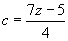What is a Linear Equation?

# ?

Key Terms

o         Linear equation

o         Constant

o         Non-linear equation

Objectives

o         Learn how to manipulate equations and still maintain the relationship between the independent and dependent variables

o         Solve linear equations for the independent variable given some specific value of the dependent variable

o         Derive a general expression for the independent variable of a linear equation

When graphing algebraic relations, you may notice that some relations plot as lines on a graph. Such relations (when the relation involves an equality) are called linear equations. A generic linear equation is shown below, where x is the independent variable, y is the dependent variable, and m and b are unspecified numbers. Note here that m and b are not variables--for a given linear equation, they can have only one value, even if it is not specified. Thus, m and b are constants rather than variables. Constants can be represented using symbols, but unlike variables, they correspond to one value only.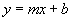Any equation that does not follow the pattern above is not a linear equation and is often called a non-linear equation. We will not review non-linear equations here, but the skills we learn do apply to non-linear equations in much the same way as they apply to linear equations.

Given a certain value for the independent variable, we know how to calculate the dependent variable for a linear equation. If we are given a value of the dependent variable, however, calculating the corresponding value of the independent variable is slightly more complicated. We will consider how to find this value (which is called solving the equation).

Primer on Manipulating Equations

Before studying how to solve linear equations, we must establish some general rules for working with equations. Consider some equation, such as that shown below.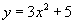Regardless of the complexity of the equation, the left-hand side is always equal to the right-hand side--this is the nature of an equation. If we make a change to either the right- or left-hand side, however, we disrupt the equality, and the two sides are no longer necessarily equal. If we make the same change to both sides, however, the equation is still valid. Let's consider why this is so. First, consider the following trivial case:

3 = 3

Obviously, this statement is true. Furthermore, if we perform the same operation or series of operations on both sides of the equation, the equation remains valid (that is, the value on one side is still equal to the value on the other side).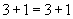(4 = 4)(15 = 15)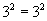(9 = 9)

The same principle applies to equations that involve variables and constants. The presence of variables simply implies that the equation is valid for more than just one particular number; nevertheless, because the two sides are equal, we can perform any series of operations on both sides and still end up with an equation. The independent and dependent variables still maintain their relationship in this case. For instance, consider the simple linear equation below.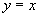The relationship between x (the independent variable) and y (the dependent variable) is maintained if we add a number to both sides of the equation.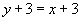For x = 3,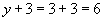Obviously, y must be 3 in this case. But since x = 3 and y = 3, we have maintained the relationship x = y. We can also see this result for multiplication: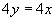If x = 2, then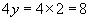Interested in learning more? Why not take an online Pre-Algebra course?

Again, y must be 2, so x = y is still valid. Thus, as long as we perform the same operation on both sides of an equation, we can make any change we like and still maintain the correct relationship between the variables.

Solving Linear Equations

We can now apply the principle we learned above to the solution of linear equations. As mentioned above, a linear equation has the following form, where x is the independent variable, y is the dependent variable, and m and b are constants.If we are given a particular value of x, we can easily calculate the corresponding value of y by simply substituting the x value and then evaluating the expression on the right-hand side of the equation. If we are given a value for y and want to calculate a corresponding value of x, however, we must take a slightly different approach. In this case, we must manipulate the equation to solve for x. Let's take a look at an example. Assume we want to calculate the value of x for the equation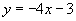when y = 7. First, substitute the value of y.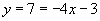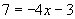Now, we can begin manipulating the equation. Our goal is to isolate x, so that we have an expression of the form x = c, where c is some number. Remember, we can perform any operation we prefer as long as we perform the same operation on both sides of the equation. Since we want to isolate x, we can start by attempting to eliminate the term –3 on the right-hand side. To do this, simply add 3 to both sides of the equation and then simplify the result.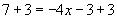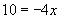Note that on the right-hand side, we are left with only the product of –4 and x. We now want to eliminate the factor –4; to do so, we can multiply by the reciprocal of –4, since the product of a number and its reciprocal is 1. Again, we must perform this operation on both sides of the equation.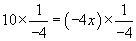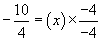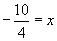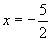At this point, we can check our answer to see if it is correct. By plugging this value of x into the equation, we should end up with the correct value of y (7, in this case).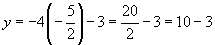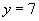Thus, our answer for the value of x is correct. The following practice problems require you to solve various linear equations for the independent variable. The process of doing so is virtually identical to the process we used above: manipulate the equation to isolate the independent variable. Remember to check your answer-this is an easy and excellent way to make sure you are doing the math correctly!

Practice Problem: For each linear equation and corresponding value of the dependent variable, calculate the value of the independent variable.

a. y = 7 - 3x, y = 16            b. y = x + 5, y = -2             c. y =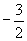x + 2, y = 4

Solution: In each case, follow the procedure illustrated in the example above. Check your answer by substituting the result back into the original equation and solving for the dependent variable. For each critical step in the solutions, a rationale is provided in parentheses to the right of the equation.

a.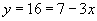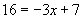(addition is commutative)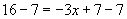(subtract 7 from both sides)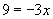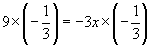(multiply both sides by the reciprocal of –3)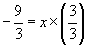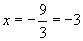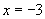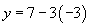(check answer)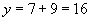(answer checks out)

b.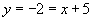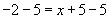(subtract 5 from both sides)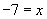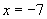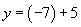(check answer)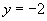(answer checks out)

c.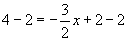(subtract 2 from both sides)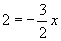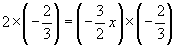(multiply both sides by the reciprocal of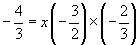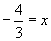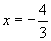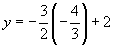(check answer)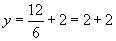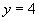(answer checks out)

A General Solution to Linear Equations

We do not necessarily need a specific value for the dependent variable to calculate an expression for the independent variable in a linear equation. For instance, we can solve the generic equationfor x without needing to know the values of any of the variables or constants. We need simply follow the procedure we used above; the task just involves using symbols (letters) instead of numbers. Let's try solving the generic linear equation for x. To isolate x, first eliminate the b term on the right-hand side by subtracting b from both sides.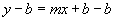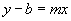Now, divide both sides by m (we can also view this as multiplying both sides by the reciprocal of m) to isolate x.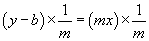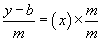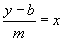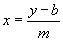We can apply this formula anytime we know y, b, and m to calculate x. For instance, in our example above, whereand y = 7, m is equal to –4, and b is equal to –3. Thus,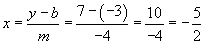Practice Problem: Solve the equation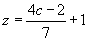for c.

Solution: Although this equation isn't currently in the standard form y = mx + b, it is still a linear equation, as we can demonstrate.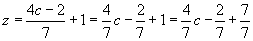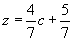We can then use the same approach as above to solve for c. Alternatively, we can use the result derived above, noting that y = z, x = c, m =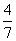, and b =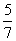.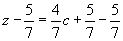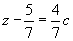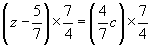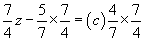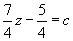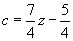or